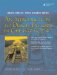# Signed and Unsigned Integral Types

This section explains the differences between signed and unsigned integral types.

The underlying binary representation of an object x of any integral type looks like this (assuming n-bit storage):

```dn-1dn-2...d2d1d0
```

where each di is either 0 or 1. The computation of the decimal equivalent value of x depends on whether x is an unsigned or signed type. If x is unsigned, the decimal equivalent value is

```dn-1*2n-1 + dn-2*2n-2 +...+ d2*22 + d1*21 + d0*20
```

The largest (positive) value that can be expressed by an unsigned integer is, therefore,

```2n - 1 = 1*2n-1 + 1*2n-2 +...+ 1*22 + 1*21 + 1*20
```

If x is signed, the decimal equivalent value is

```dn-1*-(2n-1) + dn-2*2n-2 +...+ d2*22 + d1*21 + d0*20
```

The largest (positive) value that can be expressed by a signed integer is

```2n-1 - 1 = 0*-(2n-1) + 1*2n-2 +...+ 1*22 + 1*21 + 1*20
```

This is called "two's complement" representation. To determine the representation of the negative of a signed integer,

 1 Compute the "one's complement" of the number (i.e., replace each bit with its complement). 2 Add 1 to the "one's complement" produced in the first step.

8-Bit Integer Example

Suppose that we have a tiny system that uses only 8 bits to represent a number. On this system, the largest unsigned integer would be

```11111111 = 128 + 64 + 32 + 16 + 8 + 4 + 2 + 1 = 255
```

But that same number, interpreted as a signed integer, would be

```11111111 = -128 + 64 + 32 + 16 + 8 + 4 + 2 + 1 = -1
```

Exercises: Signed and Unsigned Integral Types

You be the computer ...

For these exercises, simulate the action of the computer as it executes the given code and specify what you think the output will be. You can always compile and run the code yourself to see if your output is correct. If you disagree with the computer, try to explain why.

 1 ```#include using namespace std; int main() { unsigned n1 = 10; unsigned n2 = 9; char *cp; cp = new char[n2 n1]; if(cp == 0) cout << "That's all!" << endl; cout << "bye bye!" << endl; } ``` 2 ```#include using namespace std; int main() { int x(7), y = 11; char ch = 'B'; double z(1.34); ch += x; cout << ch << endl; cout << y + z << endl; cout << x + y * z << endl; cout << x / y * z << endl; } ``` 3 ```#include using namespace std; bool test(int x, int y) { return x / y; } int main() { int m = 17, n = 18; cout << test(m,n) << endl; cout << test(n,m) << endl; m += n; n /= 5; cout << test(m,n) << endl; } ```An Introduction to Design Patterns in C++ with Qt 4
ISBN: 0131879057
EAN: 2147483647
Year: 2004
Pages: 268